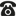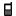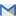## Thống kê

• truy cập   (chi tiết)
trong hôm nay
• lượt xem
trong hôm nay
• thành viên

## Hướng dẫn sử dụng thư viện

#### Lấy lại Mật khẩu trên violet.vnChào Thầy/Cô, Vì một lý do nào đó mà Thầy/Cô quên mất mật khẩu của mình trên thư viện ViOLET, Thầy/Cô có thể dùng chức năng Quên mật khẩu để lấy lại mật khẩu. (Chức năng này chỉ thực hiện được nếu khi Thầy/Cô đăng ký tài khoản có nhập số Điện thoại hoặc Email và hiện tại vẫn còn...
Xem tiếp

## Liên hệ quảng cáo

•(024) 66 745 632
•036 286 0000
•contact@bachkim.vn

## ANH 7.Nhấn vào đây để tải về
Hiển thị toàn màn hình
Báo tài liệu có sai sót
Nhắn tin cho tác giả
(Tài liệu chưa được thẩm định)
Nguồn:
Người gửi: Phan Thị Thanh Hoa
Ngày gửi: 16h:11' 02-07-2018
Dung lượng: 156.0 KB
Số lượt tải: 7
Số lượt thích: 0 người
PRACTICE THE FIRST CONGRUENCE OF TRIANGLES SIDE-SIDE-SIDE(S.S.S)

NEW WORK
+ triangle:tamgiác
+ segment: đoạnthẳng
+ arc:cungtròn
+ half-plane:nửamặtphẳng
+ we obtain: ta được
+ congruent:bằngnhau
+ corresponding angle: góctươngứng
+ measure:đo
+ measurement:sốđo
+ length:độdài
+ prove: chứngminh
+ common side:cạnhchung
+ consider:xét
+ the bisector: tiaphângiác
+ radius: bánkính
+ center: tâm
II- Exercises
Exercise 19:(Textbook-page114)
a) Consider ADE and BDE have:
AD = BD (given)
DE is common side
AE = BE (given)
ADE = BDE (S.S.S)
b) According a) we have: ADE = BDE
DAE = DBE (two corresponding angles)
Exercise 28:(workbook-page140)
Consider CAD and CBD have:
AC = BC = 3cm (given)
CD is common side
AD = BD = 2cm(given)
CAD = CBD (S.S.S)
CAD = CBD (two corresponding angles)
Exercise 32:(workbook-page141)
Consider AMB and AMC have:
AB = AC (given)
AM is common side
MB = MC (Since M is the midpointof BC)
AMB = AMC (S.S.S)
AMB = AMC (two corresponding angles)
That AMB + AMC = 1800 (two linear angles)
 AMB = AMC = 900
 AMBC at M
Exercise 3.2:(workbook-page142)
a) - Draw the segment BC = 2cm
- In the same side of the half-plane with edge BC, draw arc center B radius 3cm and arc center C radius 3cm. Two arcs above cut each other at A
- Draw the segments AB, AC, we obtain triangle ABC
b) Consider AEB and AEC have:
AB = AC = 3cm (given)
AE is common side
EB = EC (Since E is the midpoint of BC)
AEB = AEC (S.S.S)
BAE = CAE (two corresponding angles)
AE is the bisector of angle BAC
Do exercises 33,34,3.4 (Workbook-page 141,142)

PRACTICE THE SECOND CONGRUENCE OF TRIANGLES SIDE-ANGLE-SIDE(S.A.S)

NEW WORK
+ included angle: góc xen giữa
+ corollary: hệquả
+ two legs:haicạnhgócvuông
+ midpoint:trungđiểm
+Two vertical angles:haigócđốiđỉnh
+ two linear angles:haigóckềbù
+ two alternate angles:haigóc sole trong
+ exterior angle:gócngoài
+ interior angle:góctrong
+ two corresponding angles:haigócđồngvị
+ two same-side interior angles:haigóctrongcùngphía
+ two corresponding sides:haicạnhtươngứng
II- Exercises
Exercise 29:(Textbook-page 120)
We have: AC = AD + DC (D AC)
AE = AB + BE (B AE)
That AD = AB (given); DC = BE (given)
AC = AE
Consider ABC and ADE have:
AB = AD (given)
A is common angle
AC = AE (cmt)
ABC = ADE (S.A.S)
Exercise 40:(Workbook-page 142)
Consider AKM and BKM have:
AMK = BMK (= 900)
KM is common side
AM= BM (Since E is the midpoint of BC)
AKM = BKM (S.A.S)
 AKM = BKM (Two corresponding angles)
KM is the bisector of angle AKB
Exercise 42:(Workbook-page 142)
Consider ABC and DEC have:
ACB = DCE (Two vertical angles )
AC = DC (given)
BC = EC (given)
ABC = DEC (S.A.S)
 A = D (Two corresponding angles)
That A = 900 hence D = 900
Exercise 43:(Workbook-page 142)
a) Consider ABD and EBD have:
AB = EB(given )
BD is common side
ABD = EBD (since BDthe bisector of angle ABC)
ABD = EBD (S.A.S)
DA = DE (Two corresponding sides)
b) According a) we have:ABD = EBD
 A = BED (Two corresponding angles)
That A = 900 hence BED = 900
Exercise 44:(Workbook-page 142)

Gửi ý kiến